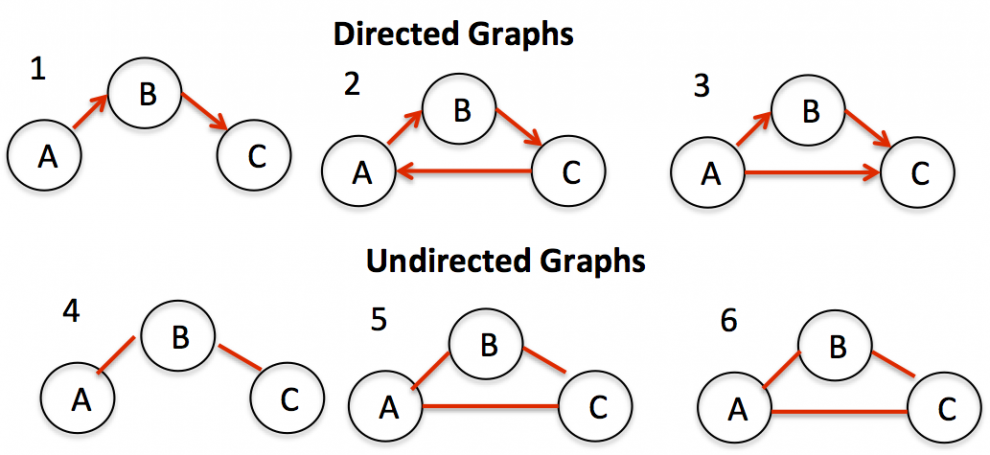Graph Cycle:

# Java Algorithm-Detect cycle in an undirected graphDetect cycle in an undirected graph-Graph cycle-The time complexity of the union-find algorithm is O(ELogV). Like directed graphs.

Given an undirected graph, how to check if there is a cycle in the graph? For example, the following graph has a cycle 1-0-2-1.We have discussed cycle detection for directed graph. We have also discussed a union-find algorithm for cycle detection in undirected graphs. The time complexity of the union-find algorithm is O(ELogV). Like directed graphs, we can use DFS to detect cycle in an undirected graph in O(V+E) time. We do a DFS traversal of the given graph. For every visited vertex ‘v’, if there is an adjacent ‘u’ such that u is already visited and u is not parent of v, then there is a cycle in graph. If we don’t find such an adjacent for any vertex, we say that there is no cycle. The assumption of this approach is that there are no parallel edges between any two vertices

Java Programming:

``````// A Java Program to detect cycle in an undirected graph
import java.io.*;
import java.util.*;

// This class represents a directed graph using adjacency list
// representation
class Graph
{
private int V;   // No. of vertices

// Constructor
Graph(int v) {
V = v;
for(int i=0; i<v; ++i)
}

// Function to add an edge into the graph
}

// A recursive function that uses visited[] and parent to detect
// cycle in subgraph reachable from vertex v.
Boolean isCyclicUtil(int v, Boolean visited[], int parent)
{
// Mark the current node as visited
visited[v] = true;
Integer i;

// Recur for all the vertices adjacent to this vertex
while (it.hasNext())
{
i = it.next();

// If an adjacent is not visited, then recur for that
if (!visited[i])
{
if (isCyclicUtil(i, visited, v))
return true;
}

// If an adjacent is visited and not parent of current
// vertex, then there is a cycle.
else if (i != parent)
return true;
}
return false;
}

// Returns true if the graph contains a cycle, else false.
Boolean isCyclic()
{
// Mark all the vertices as not visited and not part of
// recursion stack
Boolean visited[] = new Boolean[V];
for (int i = 0; i < V; i++)
visited[i] = false;

// Call the recursive helper function to detect cycle in
// different DFS trees
for (int u = 0; u < V; u++)
if (!visited[u]) // Don't recur for u if already visited
if (isCyclicUtil(u, visited, -1))
return true;

return false;
}

// Driver method to test above methods
public static void main(String args[])
{
// Create a graph given in the above diagram
Graph g1 = new Graph(5);
if (g1.isCyclic())
System.out.println("Graph contains cycle");
else
System.out.println("Graph doesn't contains cycle");

Graph g2 = new Graph(3);
if (g2.isCyclic())
System.out.println("Graph contains cycle");
else
System.out.println("Graph doesn't contains cycle");
}
}``````

Output:

```Graph contains cycle
Graph doesn't contain cycle```

Time Complexity: The program does a simple DFS Traversal of graph and graph is represented using adjacency list. So the time complexity is O(V+E)

READ  Detect Cycle in a Directed Graph#### Venkatesan Prabu

Wikitechy Founder, Author, International Speaker, and Job Consultant. My role as the CEO of Wikitechy, I help businesses build their next generation digital platforms and help with their product innovation and growth strategy. I'm a frequent speaker at tech conferences and events.

X